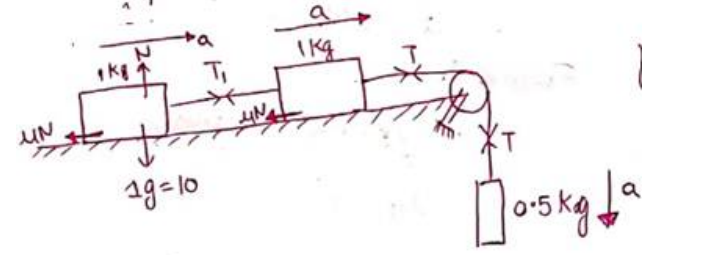Deepak Scored 45->99%ile with Bounce Back Crack Course. You can do it too!

# Solve the following :

Question:

Consider the situation shown in figure. Calculate (a) the acceleration of the $1.0 \mathrm{~kg}$ blocks, (b) the tension in the string connecting the $1.0 \mathrm{~kg}$ blocks and (c) the tension in the string attached to $0.50 \mathrm{~kg}$.

Solution:For mass $0.5 \mathrm{Kg}$

$m g-T=m a$

$0.5 g-T=0.5 a-(i)$

for $1 \mathrm{Kg}$ night mass

$T-T_{1}-\mu N=m a$

$T-T_{1}-0.2 \times 10=1 \times a$

$T-T_{1}=2+a \quad-(i i)$

For $1 \mathrm{Kg}$ left mass

$T_{1}-\mu N=m a$

$T_{1}-0.2 \times 10=1 \times a-(i i i)$

$a=0.4 \mathrm{~m} / \mathrm{s}^{2}$

$T_{1}=2.4 \mathrm{~N}$

$T_{2}=4.8 \mathrm{~N}$